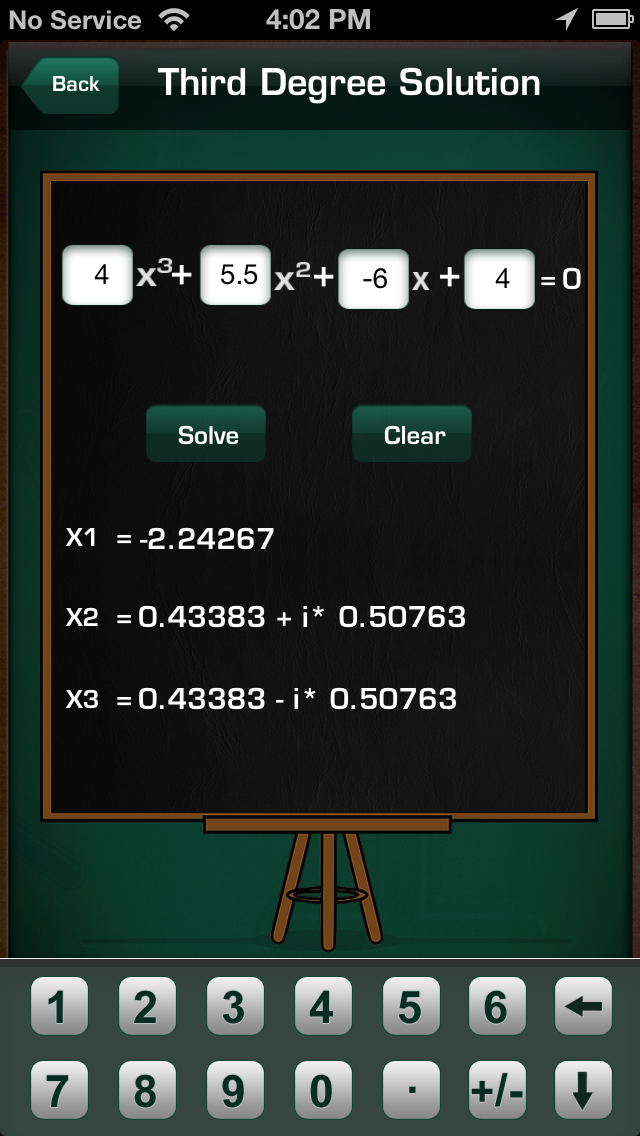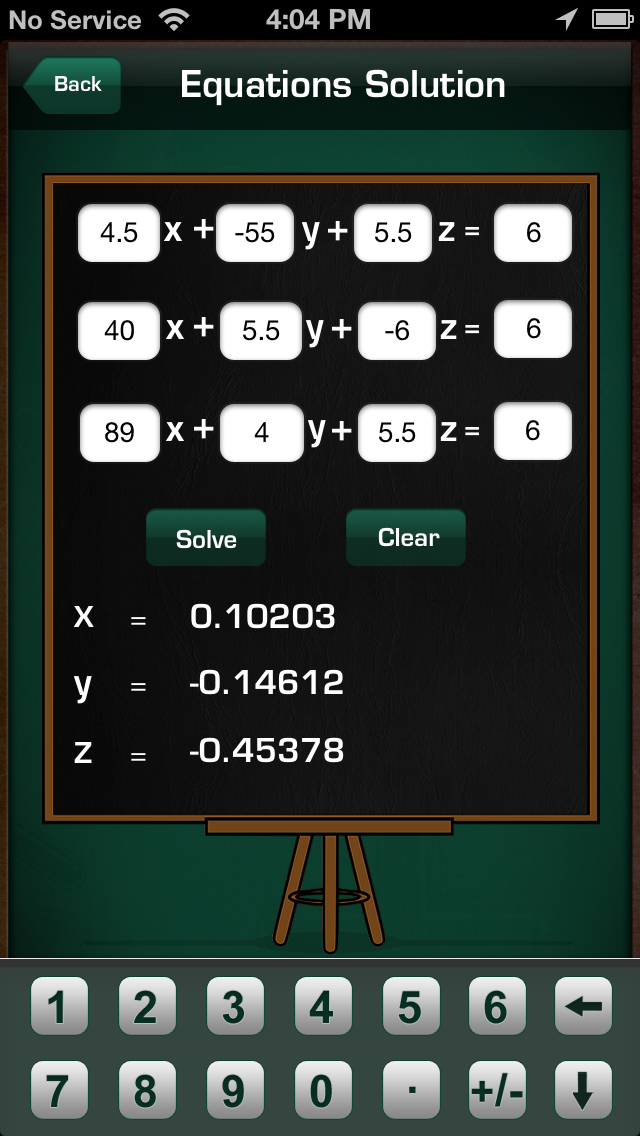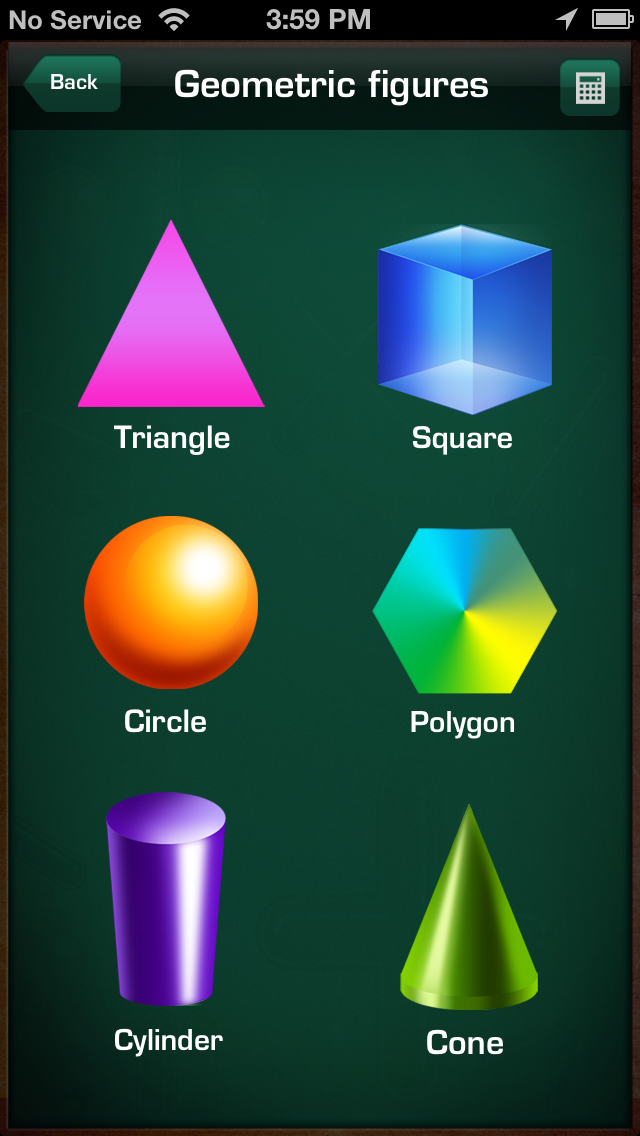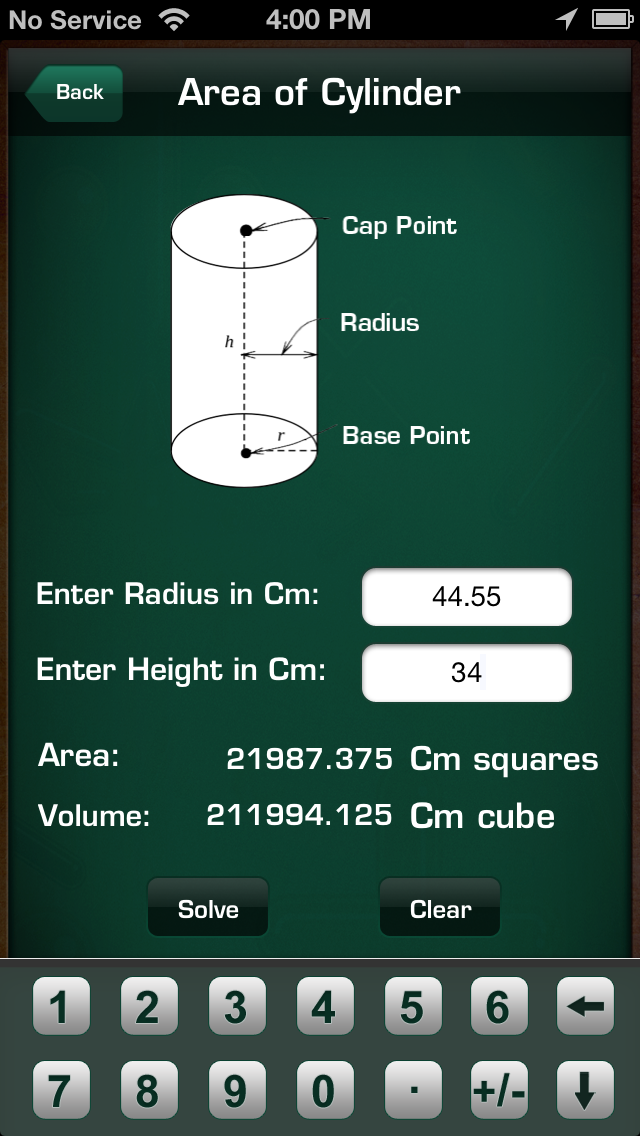## Maths Equation - Lumos Educational App StorePrice -Free

#### DESCRIPTION:

Maths Equation is a very fast and easy tool to solve quadratic equations and find area of area of 6 shapes. You input your numbers and instantaneously get solution in Fractions of second.  Excellent app for students, teachers, scientists and engineers who need an immediate and easy verification of their quadratic equations solution and area of shapes. This app can calculate the area of 6 SHAPES! With these 6 shapes, you can find the area of almost any shape. Features  ★Equations to Support  - linear equation (ax+b=c) - quadratic equation (ax^2+bx+c=0) - cubic equation (ax^3+bx^2+cx+

#### OVERVIEW:

Maths Equation is a free educational mobile app By Tatvasoft.It helps students practice the following standards .

This page not only allows students and teachers download Maths Equation but also find engaging Sample Questions, Videos, Pins, Worksheets, Books related to the following topics.

Developer URL: http://www.tatvasoft.com/iphone-applications.asp

Software Version: 1.1

Category: Education

Release Date: 2012-12-18T04:28:12Z#### Are you the Developer?

Rate this App?
3.0

2 Ratings & 2 Reviews

5
50
1
4
0
0
3
0
0
2
0
0
1
50
1EdSearch WebSearch